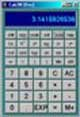# Module 1.6Resistivity Quiz

• After studying Module 1, you should be able to:
• • Descibe common materials used in electronic circuits.
• • Relate resistance to the dimensions and resistivity of a conductor.
• • Calculate the cross sectional area of conductors.
• • Carry out calculations involving resistivity & resistance.

Try a few calculations based on Resistivity. For these you just need to use the information on the Resistivity and Resistance in conductors pages. Hopefully it'll be a breeze. Because you may be using more than one formula for any problem it is important to remember to use the correct formula at the right time.

Before you start, these few tips may make the problems easier if you follow them carefully.

1. Work out the answers using pencil and paper; if you don't write out the problem you WILL get mixed up half way through and end up with the wrong answer.

2. Of course the answer is not just a number, it will be a certain number of Ohms or metres, don't forget to show the correct unit (e.g. Ω) or your answer is meaningless.

3. Convert all sub units such as mm to metres when you put them into the appropriate formula. If you slip up here you'll get really stupid answers, thousands of times too big or too small.To help you on the right track why not download our Maths Tips booklet, which shows how to use your calculator with exponents and engineering notation to deal with those sub-units and get the right answer every time.Not got a scientific calculator? The Maths Tips booklet explains what you need (and what you don't need so you don't spend your money unnecessarily). If you don't want to buy a scientific calculator, you can always pick up a free one on the net. PC users can try Calc98 from www.calculator.org/download.html. Whichever calculator you choose remember that you should read the instructions to become familiar with the working methods you should use as these do vary from calculator to calculator.

OK so now you have read these instructions, you are ready to start. Here is a way to set out a typical problem on paper so you (with practice) don't get confused.

Firstly list all the values given in the problem, followed by the value that needs to be found for the answer. For example if the problem asks for the resistance of a cable of given dimensions and material, the following list can be made:

ρ (of copper) = 1.7 x 10-8 Ωm (17 E-9 or 17 EXP-9 when entering it into your calculator in Standard Form, depending on which model you use)

L (Length of cable) = 7m

d (Diameter of cable) = 0.5mm

(500 E-6 or 500 EXP-6 metres in Standard Form)

A (Cross Sectional Area of cable in square metres) = π(d/2)2 = 3.142 x ((500 EXP-6/2)2) = 196.4 EXP-9m2

Therefore returning to the formula R = (ρL) / A

ρ = (17 EXP-9Ωm (Ohm metres)

L = 7m (metres)

and the cross sectional area A = 196.4 EXP-9m2 (square metres)

So = R = (ρL) / A = (17 EXP-9 x 7) / 196.4 EXP-9 =605mΩ (just over 0.5 ohm)

Note that when the diameter (or the radius) of a cable is given it is neccesary to firstly work out its cross sectional area in square metres (m2) before the formula relating R, to ρ, length and cross sectional area can be used. Look at the "Resistivity" page for more help in working out the cross sectional area.

Note: If you are using Calc98 for your calculations you need to set the View>Option>Display menu to Engineering (under the "Decimal" choices) and it would be a good idea whilst you are in this menu to select 2 from the Decimals drop down box, to set the number of digits after the decimal place. This will round your answer down to two decimal places, which is sufficiently accurate for most uses and stops you getting silly answers such as 4.66666666667mm, which would be far too accurate for any practical scale of measurement.

## Resistivity Calculations Practice

### 1.

A wire 12m long has a resistance of 1.5 Ω. What will be the resistance of 16m of the same wire?

### 2.

A sample of copper wire of 0.2mm radius has a length of 5m. If the resistivity of the copper is 1.7 x 10-8, what would be the resistance of the wire?

### 3.

What is the resistance of 100m of copper wire, having a diameter of 1.024mm?

### 4.

The resistance of a 50m length of wire is found to be 0.83 ohms. Its diameter is 1.15mm. What is its resistivity and from what metal is it most probably made?

### 5.

What is the length of a copper wire having a radius of 0.25mm if its resistance is found to be 0.2 Ω?

Top of Page.>